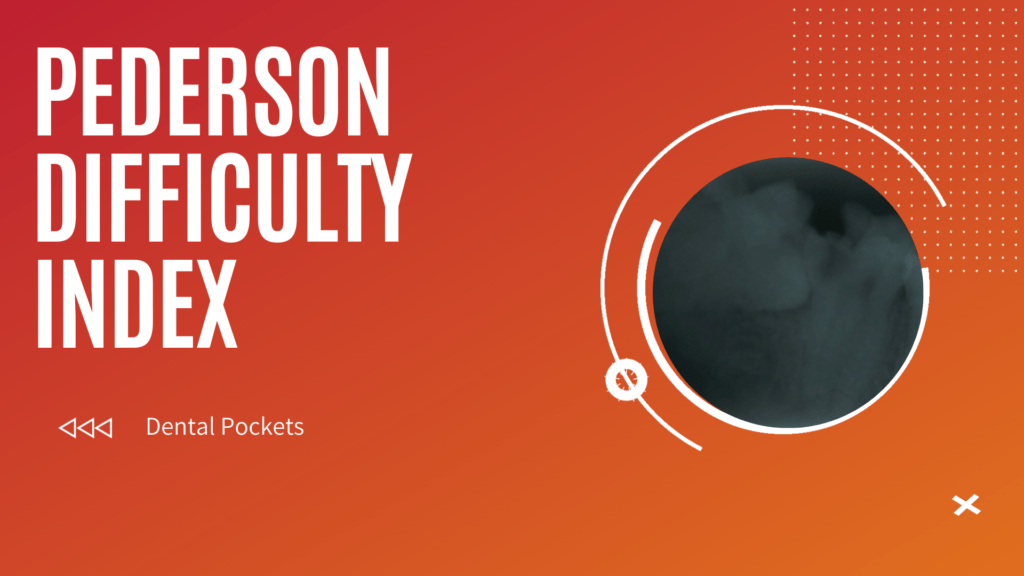Home » Oral and Maxillofacial Surgery » Pederson Difficulty Index

# Pederson Difficulty IndexPederson in 1988 proposed the difficulty index which is one of the most popular difficulty index used till date. The difficulty index is based on local anatomy observation and from radiographs. Pederson Difficulty Index

## Pederson Difficulty Index

Based on the Intraoral Periapical Radiograph or OPG, we first interpret Table 1. If the tooth is found to be Mesioangular, we add the value of 1 or if Vertical we add the value 4 or likewise. Then moving to Table 2, if the third molar is at the level of occlusal plane, we add the value of 1, if the third molar is at deep occlusal level or Level C, we add 3 or likewise. Then moving to Table 3, if there is no space between ramus and second molar, we add 3 or likewise. After adding the total of the values we get from each case, we sum the values. Based on the values we look at Table 4 and interpret the result.

Lets look at some examples.

### Example 1:

If the third molar is Vertical, Level A and Class 3, we can say that the difficulty index of that third molar is Very Difficult. Let’s see why,

Vertical: 3
Level A: 1
Class 3: 3
Total: 7 (3 + 1 + 3) => Very Difficult.

### Example 2:

If the third molar is Mesioangular, Level C and Class 1, we can say that the difficulty index of that third molar is Moderately Difficult. Let’s see why,

Mesioangular: 1
Level C: 3
Class 1: 1
Total: 5 (1 + 3 + 1) => Moderately Difficult.

### Exercise:

1. If the third molar is Distoangular, Level C, Class 3, calculate the pederson difficulty index. Mention the answer in the comments below.
2. If the third molar is Vertical, Level A, Class 2, calculate the pederson difficulty index. Mention the answer in the comments below.

I hope this clears any doubts you have regarding the Pederson Difficulty Index (1988).

## References

1. Pederson GW. Surgical removal of tooth. In: Pederson GW, editor. Oral surgery. Philadelphia: WB Saunders; 1988.
2. Malik NA. Text book of oral and maxillofacial surgery. New Delhi: Jay pee brothers medical publisher; 2008.